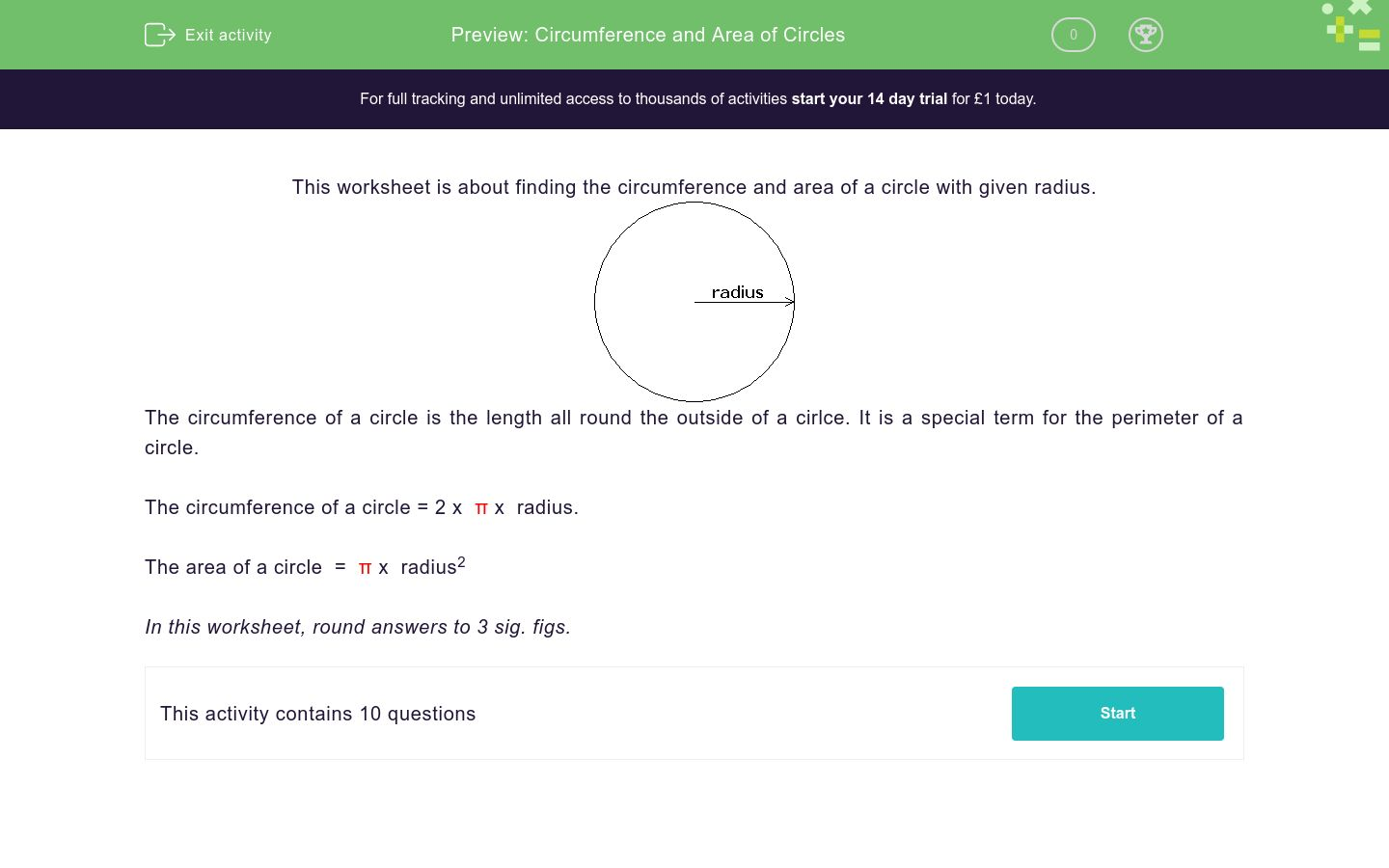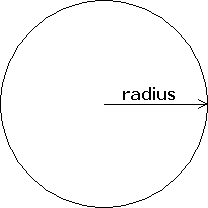# Circumference and Area of Circles

In this worksheet, students find the circumference and area of the circles with given radii.Key stage:  KS 4

Curriculum topic:  Geometry and Measures

Curriculum subtopic:  Identify and Apply Circle Definitions and Properties

Difficulty level:### QUESTION 1 of 10

This worksheet is about finding the circumference and area of a circle with given radius.The circumference of a circle is the length all round the outside of a cirlce. It is a special term for the perimeter of a circle.

The circumference of a circle = 2 x  π x  radius.

The area of a circle  =  π x  radius2

In this worksheet, round answers to 3 sig. figs.

Find the circumference of a circle with radius 5 cm

Just write the number in the box below. Don't forget to round to 3 sig. figs.

Find the circumference of a circle with radius 16 cm

Just write the number in the box below. Don't forget to round to 3 sig. figs.

Find the circumference of a circle with radius 14.2 m

Just write the number in the box below. Don't forget to round to 3 sig. figs.

Find the circumference of a circle with radius 40.7 m

Just write the number in the box below. Don't forget to round to 3 sig. figs.

Find the area of a circle with radius 40.7 m

Just write the number in the box below. Don't forget to round to 3 sig. figs.

Find the area of a circle with radius 14.2 m

Just write the number in the box below. Don't forget to round to 3 sig. figs.

Find the area of a circle with radius 16 cm

Just write the number in the box below. Don't forget to round to 3 sig. figs.

Find the area of a circle with radius 5 cm

Just write the number in the box below. Don't forget to round to 3 sig. figs.

Find the circumference of a circle with radius 5.6 cm

Just write the number in the box below. Don't forget to round to 3 sig. figs.

Find the area of a circle with radius 5.6 cm

Just write the number in the box below. Don't forget to round to 3 sig. figs.

• Question 1

Find the circumference of a circle with radius 5 cm

Just write the number in the box below. Don't forget to round to 3 sig. figs.

CORRECT ANSWER
31.4
EDDIE SAYS
2 × π × 5
• Question 2

Find the circumference of a circle with radius 16 cm

Just write the number in the box below. Don't forget to round to 3 sig. figs.

CORRECT ANSWER
101
EDDIE SAYS
2 × π × 16
• Question 3

Find the circumference of a circle with radius 14.2 m

Just write the number in the box below. Don't forget to round to 3 sig. figs.

CORRECT ANSWER
89.2
EDDIE SAYS
2 × π × 14.2
• Question 4

Find the circumference of a circle with radius 40.7 m

Just write the number in the box below. Don't forget to round to 3 sig. figs.

CORRECT ANSWER
256
EDDIE SAYS
2 × π × 40.7
• Question 5

Find the area of a circle with radius 40.7 m

Just write the number in the box below. Don't forget to round to 3 sig. figs.

CORRECT ANSWER
5200
EDDIE SAYS
π × 40.7²
• Question 6

Find the area of a circle with radius 14.2 m

Just write the number in the box below. Don't forget to round to 3 sig. figs.

CORRECT ANSWER
633
EDDIE SAYS
π × 14.2²
• Question 7

Find the area of a circle with radius 16 cm

Just write the number in the box below. Don't forget to round to 3 sig. figs.

CORRECT ANSWER
804
EDDIE SAYS
π × 16²
• Question 8

Find the area of a circle with radius 5 cm

Just write the number in the box below. Don't forget to round to 3 sig. figs.

CORRECT ANSWER
78.5
EDDIE SAYS
π × 5²
• Question 9

Find the circumference of a circle with radius 5.6 cm

Just write the number in the box below. Don't forget to round to 3 sig. figs.

CORRECT ANSWER
35.2
EDDIE SAYS
2 × π × 5.6
• Question 10

Find the area of a circle with radius 5.6 cm

Just write the number in the box below. Don't forget to round to 3 sig. figs.

CORRECT ANSWER
98.5
EDDIE SAYS
π × 5.6²
---- OR ----

Sign up for a £1 trial so you can track and measure your child's progress on this activity.

### What is EdPlace?

We're your National Curriculum aligned online education content provider helping each child succeed in English, maths and science from year 1 to GCSE. With an EdPlace account you’ll be able to track and measure progress, helping each child achieve their best. We build confidence and attainment by personalising each child’s learning at a level that suits them.

Get started#### Similar activities you may like

Start your £1 trial today.
Subscribe from £10/month.